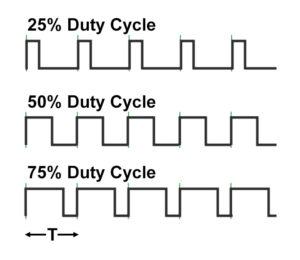# LED Dimming Using the PULSE Option and PWM

With the PULSE option for the SFA base you can use an external controller (computer, function generator, etc.) to turn the LED on and off at full intensity with precise timing. In addition you can use PWM (pulse width modulation) as a dimmer control. With PWM you input a square wave signal at a fixed frequency f (period T = 1/f). The relative amount of ON time vs OFF time (the duty cycle) during each period determines the brightness of the output. The shorter the on time, the dimmer the light. The figure below shows the difference between 25%, 50%, and 70% duty cycles.You can compute the relative output intensity (dimming level) with this formula:

DIM = Df *6×10-4

where:

• DIM is the dimming level, expressed as a fraction (e.g., 50% = 0.5)
• D is the duty cycle, expressed as a fraction (e.g., 50% = 0.5)
• f is the square wave frequency, in Hertz (Hz)

As an example, for a duty cycle D of 50% and a square wave frequency f of 150 Hz, we get

DIM = 0.5 – 150*6*10-4 = 0.5 – 0.09 = 0.41, or 41% intensity

Conversely, if you have a desired dimming level you can compute the duty cycle needed for any given frequency:

D = DIM + f*6×10-4

As an example, for a desired DIM level of 50% intensity and a frequency of 150 Hz, we get:

D = 0.5 +150*6*10-4 = 0.5 + 0.09 = 0.59, or 59% duty cycle

The properties of the electronic circuit in the SFA impose certain constraints:

• Voltage level: 2.8 – 6.0 VDC for ON, <0.6VDC OFF
• Maximum square wave frequency: 200 Hz
• Minimum ON time (D/f): 0.7msec

For this last constraint you can compute the minimum permissible duty cycle for any given frequency:

D = f*.0007

As an example, for a frequency of 150 Hz the minimum duty cycle is:

D = 150 * .0007 = 0.105, or 10.5%

The corresponding dimming level would be:

DIM = 0.105 – 150*6*10-4 = 0.105 – 0.09 = 0.015, or 1.5% intensity

Contact NIGHTSEA if you need any additional information.